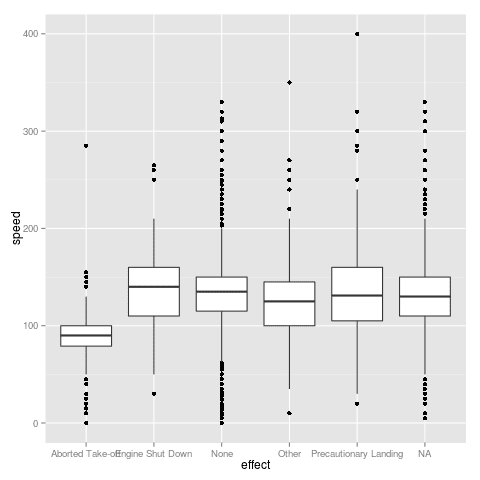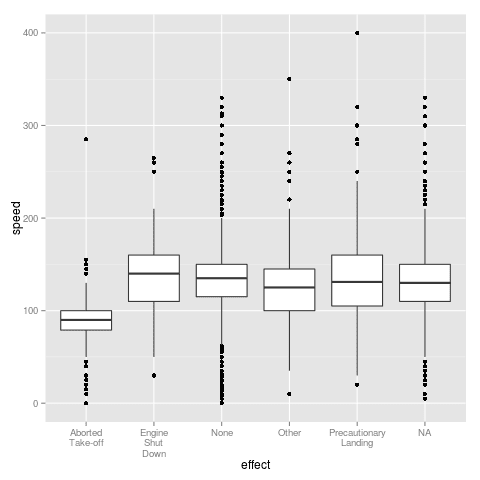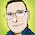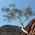## Thursday, October 17, 2013

### Line Breaks Between Words in Axis Labels in ggplot in R

Sometimes when plotting factor variables in R, the graphics can look pretty messy thanks to long factor levels. If the level attributes have multiple words, there is an easy fix to this that often makes the axis labels look much cleaner.

### Without Line Breaks

Here's the messy looking example:No line breaks in axis labels
And here's the code for the messy looking example:

```library(OIdata)
data(birds)
library(ggplot2)

ggplot(birds,
aes(x = effect,
y = speed)) +
geom_boxplot()
```

### With Line Breaks

We can use regular expressions to add line breaks to the factor levels by substituting any spaces with line breaks:

```library(OIdata)
data(birds)
library(ggplot2)

levels(birds\$effect) <- gsub(" ", "\n", levels(birds\$effect))
ggplot(birds,
aes(x = effect,
y = speed)) +
geom_boxplot()
```Line breaks in axis labels
Just one line made the plot look much better, and it will carry over to other plots you make as well. For example, you could create a table with the same variable.

### Horizontal Boxes

Here we can see the difference in a box plot with horizontal boxes. It's up to you to decide which style looks better:No line breaks in axis labelsLine breaks in axis labels

```library(OIdata)
data(birds)
library(ggplot2)

levels(birds\$effect) <- gsub(" ", "\n", levels(birds\$effect))
ggplot(birds,
aes(x = effect,
y = speed)) +
geom_boxplot() +
coord_flip()
```

Just a note: if you're not using ggplot, the multi-line axis labels might overflow into the graph.

The code is available in a gist.

### Citations and Further Reading

1.You could also break using a set character width so that a line break is not necessarily added for every space. That is, you want a maximum width of 25 characters:

sapply(strwrap(as.character(value), width=25, simplify=FALSE), paste, collapse="\n")

You can steal our function in the likert package: https://github.com/jbryer/likert/blob/master/R/label_wrap_mod.r

2.This is helpful, thanks Mollie, and to Jason for the alternative. I'll try them both out. Though Mollie, your first two code fragments are the same are they not? I imagine that the gsub line should not be in your 'messy example'?

3.Thanks, David! I fixed it.